## RS Aggarwal Class 7 Solutions Chapter 3 Decimals Ex 3B

These Solutions are part of RS Aggarwal Solutions Class 7. Here we have given RS Aggarwal Solutions Class 7 Chapter 3 Decimals Ex 3B.

Other Exercises

Question 1.
Solution:
Converting them into like decimals 16.00, 8.70, 0.94, 6.80 and 7.77
16.0 + 8.70 + 0.94 + 6.80 + 7.77 = 40.21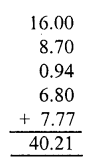Question 2.
Solution:
Converting them into like decimals 18.600, 206.370, 8.008, 26.400, 6.900
18.600 + 206.370 + 8.008 + 26.400 + 6.900 = 266.278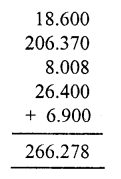Question 3.
Solution:
Converting them into like decimals, 63.50, 9.70, 0.80, 26.66, 12.17
63.50 + 9.70 + 0.80 + 26.66 + 12.17 = 112.83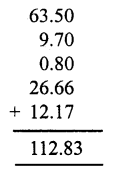Question 4.
Solution:
Converting them into like decimals 17.400, 86.390, 9.435, 8.800, 0.060
17.400 + 86.390 + 9.435 + 8.800 + 0.060 = 122.085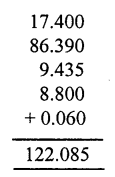Question 5.
Solution:
Converting them into like decimals 26.900, 19.740, 231.769, 0.048
26.900 + 19.740 + 231.769 + 0.048 = 278.457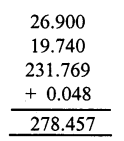Question 6.
Solution:
Converting them into like decimals 23.800, 8.940, 0.078 and 214.600
23.800 + 8.940 + 0.078 + 214.600 = 247.418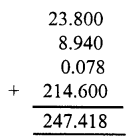Question 7.
Solution:
Converting them into like decimals.
6.606, 66.600, 666.000,0.066, 0.660
6.606 + 66.600 + 666.000 + 0.066 + 0,660 = 739.932Question 8.
Solution:
9.090, 0.909, 99.900, 9.990, 0.099
9.090 + 0.909 + 99.900 + 9.990 + 0.099 = 119.988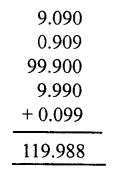Subtract:
Question 9.
Solution:
14.79 from 72.43
72.43 – 14.79 = 57.64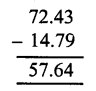Question 10.
Solution:
Converting them into like decimals, We get
36.74 and 52.60
Now 52.60 – 36.74 = 15.86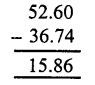Question 11.
Solution:
Converting them into like decimals, We get
13.876 and 22.000
22.000 – 13.876 = 8.124Question 12.
Solution:
Converting them into like decimals, We get
15.079 and 24.160
24.160 – 15.079 = 9.081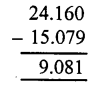Question 13.
Solution:
Converting them into like decimals We get
0.680 and 1.007
1.007 – 0.680 = 0.327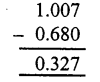Question 14.
Solution:
Converting them into like decimals,
We get 0.4678 and 5.0500
5.0500 – 0.4678 = 4.5822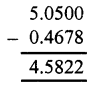Question 15.
Solution:
Converting them into like decimals,
We get 2.5307 and 8.0000
8.0 – 2.5307 = 5.4693Question 16.
Solution:
There are like decimals
9.1 – 6.732 = 2.269Question 17.
Solution:
Converting them into like decimals,
We get 5.746 and 9.100
9.100 – 5.746 = 3.354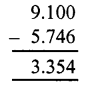Question 18.
Solution:
Converting into like decimals, we get,
63.59 and 92.00
Required number = 92.00 – 63.58 = 28.42Question 19.
Solution:
Converting into like decimals, we get:
8.100 and 0.813
Required number = 8.100 – 0.813 = 7.287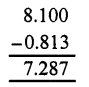Question 20.
Solution:
Converting them into like decimals, we get: 32.67 and 60.10
Required number = 60.10 – 32.67 = 27.43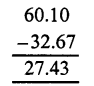Question 21.
Solution:
Converting into like decimals, we get 74.3 and 26.87
Required number = 74.30 – 26.87 = 47.43Question 22.
Solution:
Cost of notebook = Rs. 23.75
Cost ofpencil = Rs. 2.85
Costofpen =Rs. 15.90
Total cost = Rs. 42.50
Amount gave to the shop keeper = 50 rupees
Balance amount got = Rs 50.00 – Rs 42.50 = 7.50

Hope given RS Aggarwal Solutions Class 7 Chapter 3 Decimals Ex 3B are helpful to complete your math homework.

If you have any doubts, please comment below. Learn Insta try to provide online math tutoring for you.